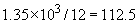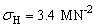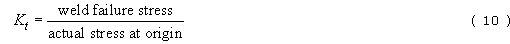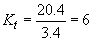Introduction to forensic engineering

Start this free course now. Just create an account and sign in. Enrol and complete the course for a free statement of participation or digital badge if available.

Free course

# 6.6 Weld defects

Although weld strengths appeared reasonably high, there was a small difference of about 4 per cent between the tensile strength of welds from intact and cracked panels. Could mechanical analysis be used to check the stress concentrating effect of the pinholes? The first point to check was the formula needed to calculate the hoop stress in the vessel. Hoop stress acts around the circumference of a tank, or pressure vessel.

Fortunately, the stress situation in a static pressure vessel is simple. Where the ratio of tank radius to wall thickness is greater than about 10, the tank can be treated as a thin-walled pressure vessel. The ratio is in factand the hoop stress, σH is given by Equation (7), Box 19.

Knowing r and t, and calculating p using Equation (9) with a known density, the hoop stress at the failure crack was evaluated asIt is now possible to calculate the stress concentration that would have been acting at the critical pinhole, shown in Figure 5, Paper 4, prior to the first growth of the crack. Because from Equation (3)HenceFrom the mechanical tests of welds, it was possible to calculate that the real stress acting at the pinhole in the centre of the lower single-thickness panel was about six times the hoop stress from the hydrostatic pressure of the contents. A cross check on the figure is possible from SAQ 20, where Kt was estimated at about 14 times the nominal applied stress. As mentioned in the SAQ, there is some uncertainty in the assumed diameter of the hole, which could cause a large error in the estimate.